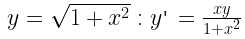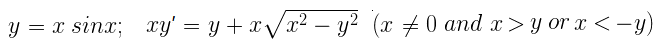NCERT Solutions for Maths Class 12 Exercise 9.2

# NCERT Solutions for Maths Class 12 Exercise 9.2

## NCERT Solutions for Maths Class 12 Exercise 9.2

Hello Students. Welcome to maths-formula.com. In this post, you will find the complete NCERT Solutions for Maths Class 12 Exercise 9.2.

You can download the PDF of NCERT Books Maths Chapter 9 for your easy reference while studying NCERT Solutions for Maths Class 12 Exercise 9.2.

Class 12th is a very crucial stage of your student’s life, since you take all important decisions about your career on this stage. Mathematics plays a vital role to take decision for your career because if you are good in mathematics, you can choose engineering and technology field as your career.

NCERT Solutions for Maths Class 12 Exercise 9.2 helps you to solve each and every problem with step by step explanation which makes you strong in mathematics.

All the schools affiliated with CBSE, follow the NCERT books for all subjects. You can check your syllabus from NCERT Syllabus for Mathematics Class 12.

NCERT Solutions for Maths Class 12 Exercise 9.2 are prepared by the experienced teachers of CBSE board. If you are preparing for JEE Mains and NEET level exams, then it will definitely make your foundation strong.

If you want to recall All Maths Formulas for Class 12, you can find it by clicking this link.

If you want to recall All Maths Formulas for Class 11, you can find it by clicking this link.

NCERT Solutions for Maths Class 12 Exercise 9.6

## NCERT Solutions for Maths Class 12 Exercise 9.2

In each of the Exercises 1 to 10, verify that the given functions (explicit or implicit) is a solution of the corresponding differential equation.

Maths Class 12 Ex 9.2 Question 1.

y = ex + 1       :         y’’ – y’ = 0

Solution:

The given function is y = ex + 1          … (i)

Differentiating equation (i) w.r.t. x, we get

y’ = ex     … (ii)                    and                  y’’ = ex        … (iii)

Subtracting equation (ii) from equation (iii), we get y’’ – y’ = 0

Hence, the function y = ex + 1 is the solution of y’’ – y’ = 0.

Maths Class 12 Ex 9.2 Question 2.

y = x2 + 2x + C       :         y’ – 2x – 2 = 0

Solution:

The given function is y = x2 + 2x + C          … (i)

Differentiating equation (i) w.r.t. x, we get

y’ = 2x + 2                       y’ – 2x – 2 = 0       … (ii)

Hence, the function y = x2 + 2x + C is the solution of y’ – 2x – 2 = 0.

Maths Class 12 Ex 9.2 Question 3.

y = cos x + C       :         y’ + sin x = 0

Solution:

The given function is y = cos x + C          … (i)

Differentiating equation (i) w.r.t. x, we get

y’ = –sin x                      y’ + sin x = 0      … (ii)

Hence, the function y = cos x + C is the solution of y’ + sin x = 0.

Maths Class 12 Ex 9.2 Question 4.Solution:

The given function is y = √(1 + x2)      … (1)

Differentiating equation (i) w.r.t. x, we get

Hence, the function y = √(1 + x2) is the solution of y’ = xy/(1 + x2).

Maths Class 12 Ex 9.2 Question 5.

y = Ax       :         xy’ = y     (x ≠ 0)

Solution:

The given function is y = Ax          … (i)

Differentiating equation (i) w.r.t. x, we get

y’ = A               … (ii)

Dividing equation (ii) by equation (i), we get

y’/y = 1/x         xy’ = y

Hence, the function y = Ax is the solution of xy’ = y (x ≠ 0).

Maths Class 12 Ex 9.2 Question 6.Solution:

The given function is y = x sin x          … (i)

Differentiating equation (i) w.r.t. x, we get

y’ = x cos x + sin x               … (ii)

Multiplying equation (ii) by x, we get

xy’ = x2 cos x + x sin x

Maths Class 12 Ex 9.2 Question 7.

Solution:

The given function is xy = log y + C          … (i)

Differentiating equation (i) w.r.t. x, we get

Maths Class 12 Ex 9.2 Question 8.

y – cos y = x       :        (y sin y + cos y + x) y’ = y

Solution:

The given function is y – cos y = x          … (i)

Differentiating equation (i) w.r.t. x, we get

y’ – (–sin y) y’ = 1                 y’(1 + sin y) = 1      … (ii)

Multiplying equation (ii) by y, we get

yy(1 + sin y) = y                 y’(y + y sin y) = y                 y’(x + cos y + y sin y) = y

Hence, the function y – cos y = x is the solution of y’(x + cos y + y sin y) = y.

Maths Class 12 Ex 9.2 Question 9.

x + y = tan-1 y       :        y2 y + y2 + 1 = 0

Solution:

The given function is x + y = tan-1 y          … (i)

Differentiating equation (i) w.r.t. x, we get

1 + y’ = 1/(1 + y2) × y                  (1 + y’) (1 + y2) = y

1 + y2 + y’ + y2 y’ = y                y2 y + y2 + 1 = 0       … (ii)

Hence, the function x + y = tan-1 y is the solution of y2 y + y2 + 1 = 0.

Maths Class 12 Ex 9.2 Question 10.

Solution:

Maths Class 12 Ex 9.2 Question 11.

The number of arbitrary constants in the general solution of a differential equation of fourth order are:
(A) 0
(B) 2
(C) 3
(D) 4

Solution:

(D) The general solution of a differential equation of fourth order has 4 arbitrary constants.
Because it contains the same number of arbitrary constants as the order of differential equation.

Maths Class 12 Ex 9.2 Question 12.

The number of arbitrary constants in the particular solution of a differential equation of third order are:
(A) 3
(B) 2
(C) 1
(D) 0

Solution:

(D) Number of arbitrary constants = 0
Because particular solution is free from arbitrary constants.

NCERT Solutions for Maths Class 12 Exercise 9.6